Платформа: Все платформы Версии: Все версии

## Описание проблемы

Stationary (time-invariant) models with nonlinearities may converge very slowly. A nonlinearity can be introduced into the model either in the governing equation, or by making any of the material properties, loads, or boundary conditions dependent upon the solution. Multiphysics problems are often nonlinear. If instead the model is linear, see: Knowledgebase 1260: What to do when a linear stationary model is not solving. This guide applies solely to nonlinear stationary models.

## Решение

The issue here has do with the iterative algorithm used to solve nonlinear stationary models. The algorithm is, generally speaking, a Newton's method approach. That is, when solving, the software starts with the user-specified initial values to evaluate all solution-dependent terms. The software then computes an initial solution and from there it iteratively re-computes the solution, taking into account how these intermediate solutions affect the nonlinearities. When the difference in the computed solutions between successive iterations is sufficiently small, or when the residual is sufficiently small, the problem is considered converged to within the specified tolerance. Assuming a well-posed problem, the solver may converge slowly (or not at all) if the initial values are poor, if the nonlinear solver is not able to approach the solution via repeated iterations, or if the mesh is not fine enough to resolve the spatial variations in the solution.

#### Initial Values

The default Initial Values for the unknowns in most physics interfaces are zero. The exceptions are the Heat Transfer interfaces, which have a default Initial Value of 293.15K, or 20°C, for the temperature fields. Convergence can be poor when the initial values do not provide a good starting point for this iterative approach. If a good estimate to the solution field is known, this can be entered as an an expression in the Initial Value field. With the exception of some thermal problems however, it is often difficult to estimate the solution, so alternative approaches are needed.

One can say that, in general, if the loads on a nonlinear system are zero, the system will be at rest; that is, the solution will be zero. Therefore, an initial value of zero is almost always reasonable if a very small load is applied. Starting from zero initial conditions, the nonlinear solver will most likely converge if a sufficiently small load is applied. That is, start by first solving a model with a small, but non-zero, load. From there, if an additional small load increment is applied, the previously computed solution is a reasonable initial condition. Extending this logic, if one wants to solve for any arbitrary load on a nonlinear system, it makes sense to solve a sequence of intermediate problems with gradually increasing load values and using the solutions from each previous step as the initial condition for the next step. This approach is known as a Continuation Method with a Constant predictor. The Continuation method is enabled by default when using the Auxiliary sweep study extension, as shown below.The Auxiliary Sweep can be used to implement ramping of any Global Parameter.

A Global Parameter has to be introduced (in the above screenshot, P) and is ramped from a value nearly zero up to one. This parameter is used within the physics interfaces to multiply one, some, or all of the applied loads.

Using a predictor of type Constant will take the solution from the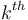iteration and use it as the initial value for the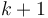iteration. That is: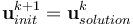It is also possible to compute the derivative of the solution with respect to the continuation parameter and use that derivative (evaluated at theiteration) to compute a new initial value: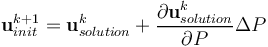where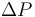is the stepsize of the continuation parameter. The latter method is known as the Continuation Method with a Linear predictor, and is controlled within the Study Configurations as shown in the screenshot below.The settings controlling the predictor type.

The Automatic predictor setting will use the constant predictor when a segregated solution approach is being used, and use the linear predictor when the fully coupled approach is used. The fully coupled and segregated approaches are discussed below.

The advantages of the continuation method are two-fold. First, it is physically intuitive, often matching how one would perform an experiment. Second, the continuation method will automatically take smaller load increments if a solution cannot be found. For example, if ramping `P` over values of: `0.2,0.4,0.6,0.8,1.0` the nonlinear solver may fail to converge for a value of `0.8`. In that case, the continuation method will automatically backtrack and try to solve for intermediate values in the range of `0.6` through `0.8`. This is useful since the software will then return an estimation of the maximum possible loadcase for which the solver can converge.

#### Nonlinearity Ramping

The technique of load ramping is not always reasonable for all problems. In such cases, use the same continuation method, but instead ramp the nonlinearities in the model. Nonlinearities arise as a consequence of the governing equation, as a material nonlinear expression, or as a coupling term between physics. Again, introduce a Global Parameter that gets ramped from exactly zero to one. Use this parameter to modify the nonlinearity expressions in the model. For example, if there is a temperature-dependent material property such as:
``` k(T) = 10[W/m/K]*exp(-(T-293[K])/100[K]) ```
replace it with the expression:
``` k(T,P) = 10[W/m/K]*((1-P)+P*exp(-(T-293[K])/100[K])) ```
At a value of `P=0` the above expression is linear, and at a value of `P=1` the expression is equal to the original nonlinear expression. As P is ramped up, the continuation method uses the previous solutions to compute initial conditions for the more nonlinear cases. If you define this nonlinearity ramping such that the first case `(P=0)` is a purely linear problem, then you are guaranteed to get a solution for this first step in the ramping.

Nonlinearity ramping is an especially useful technique if any of the nonlinear terms in the model are very abrupt. In the extreme case, suppose one wants to model an instantaneous change in properties, such as:
``` k(T) = 10[W/m/K]+10[W/m/K]*(T>400[K]) ```
That is, the material property changes instantaneously from `10W/m/K` to `20W/m/K` at `400K`. This case is generally difficult, or impossible, to solve since this material property is non-smooth. Instead, use a nonlinear material property expression that ramps from a very smooth function to a very nearly discontinuous one. The continuation method will again backtrack and try intermediate values of the ramping parameter, thus giving you the nearest approximation to the abrupt transition that is solvable.

Load ramping and nonlinearity ramping can be used in combination, but start with only one or a few of the loads or nonlinearities being ramped. Check the solver log to see if the continuation method is backtracking. If it does so, use a finer increment in that range.

#### Mesh Refinement

If both load ramping and nonlinearity ramping are still leading to slow convergence, refine the mesh. The finite element mesh must be fine enough to resolve the spatial variations in the solution fields. Ideally, one would use small elements in regions where the solution varies quickly in space, and larger elements elsewhere. However, it is usually not possible to know this ahead of time. Therefore, it is recommended to use Adaptive Mesh Refinement which will automatically refine the mesh only in regions where it is needed, and coarsen the mesh elsewhere. It is also possible to manually refine the mesh. For more details, see: Performing a Mesh Refinement Study

Mesh refinement may often need to be combined with load or nonlinearity ramping and may require a set of studies, first starting with a relatively coarse mesh for nonlinearity ramping, refining the mesh, and the ramping further on the refined mesh. An example model that combines the techniques of nonlinearity ramping and adaptive mesh refinement with multiple study steps is: Cooling and Solidification of Metal

#### Solution Approach

There are two approaches that can be used when iteratively solving the nonlinear system of equations: a Fully Coupled or a Segregated approach. The former approach solves for all unknowns in the problem at once, and considers all coupling terms between all unknowns within a single iteration. This is relatively expensive to do, but will lead to the most robust convergence. This approach is used by default for most 1D, 2D, and 2D-axisymmetric models.

The segregated approach, on the other hand, solves sets of unknowns separately. The unknowns are segregated into groups, usually according the physics that they represent, and these groups are solved one after another. That is, within each outer Newton-type iteration, the segregated approach solves for each segregated group sequentially. Each physics is thus solved as a standalone problem, using the solution from any previously computed steps as initial values and linearization points. The coupling terms between the different groups are thus neglected. Despite this, the segregated approach can often converge very robustly, unless there are very strong couplings between the physics in the model. The memory requirements will always be lower than with the fully coupled approach, and the overall solution time can often be lower as well. This segregated approach is used by default for most 3D multiphysics models, and the software will automatically segregate the problem into appropriate groups.

To switch between these solver types, go to the Stationary Solver node within the Study sequence. There will always already be either a Segregated or Fully Coupled feature beneath this. Right-click on the Stationary Solver node and add either the Segregated or Fully Coupled feature. Within either of these features, it can also be helpful to enable the Results While Solving option, as shown in the screenshot below, to visualize the iterations being taken during the solution.The Fully Coupled solution approach, with the Plot While Solving enabled.

It is sometimes necessary to manually scale the dependent variables. See Knowledge Base 1240: Manually Setting the Scaling of Variables

The other low-level default settings within the Stationary Solver are chosen for robustness. That is, they are tuned to achieve convergence in as many cases as possible. It is quite rare that changing these settings is superior to using a combination of the other techniques in this Knowledgebase, although it is possible to tune these settings to reduce solution time and memory requirements, once a model is already converging. Changes to these low-level settings from the defaults will usually be quite model- and case-specific.

#### General Approach to Model Checking

If it is not clear that any of the above strategies are working, it is useful to take a more general approach to verifying the general validity of the model. This involves a systematic reduction in the model complexity. Examine the model and identify all terms that introduce nonlinearities, such as multiphysics couplings, nonlinear materials relationships, and nonlinear boundary conditions. With respect to any nonlinearities, replace them by a reasonable linearized term. Repeat this for every nonlinearity of the model. With respect to multiphysics couplings, rather than solving the problem using a fully coupled approach (the default) solve the problem sequentially, with one physics being solved after another. The objective here is to simplify the model to a state where the model will solve, with linear approximations. With sufficient simplification, a model can be reduced to a linear problem, and if this simplified model does not converge, see: What to do when a linear stationary model is not solving.

Once a simplified solvable version of the model has been found, gradually increase the model complexity again, re-introducing nonlinearities and multiphysics couplings. Using this technique systematically, along with the techniques described previously, will usually identify the nonlinearities in the model that are leading to issues. It may also reveal that the model itself is ill-posed in some way. Sometimes, reducing the model complexity can be quite challenging and it can be better to start from as simple a case as possible and gradually increase the complexity. It is thus always advised to start this procedure with a simplified 2D, or 2D-axisymmetric model.

#### Converting to a Time-Dependent Formulation

If all of the above approaches have been tried and you are certain that the problem itself is well-posed, consider that the nonlinear problem may not, in fact, have a stationary (time-invariant) solution. A classic example of this is fluid flow around a cylinder with high, but constant, flow rates. At low flow speeds the flow solution will be time invariant, but at higher flow rates there will be vortex shedding, a time-varying change in the flow field behind the cylinder. Such problems must solved in the time domain.

In such cases it will be particularly helpful to ramp the load gradually in time, from consistent initial values. Ramping the nonlinearities over time is not as strongly motivated, but step changes in nonlinearities should be smoothed out throughout the simulation. Use either a very fine mesh throughout the simulation domain or use adaptive mesh refinement.